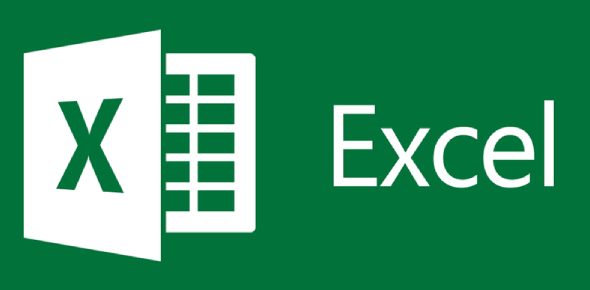# Basic Excel Quiz Questions: MCQ!

10 Questions | Total Attempts: 5221SettingsMicrosoft Excel is a spreadsheet program for creating and editing spreadsheets saved with a. Xls or. Xlsx file extension. MS Excel is widely used for data processing and financial modeling. To become an expert, one needs to master the basic formulas and functions used in the excel spreadsheet. This quiz will test your knowledge about the basic formulas and functions of Excel.

• 1.
Number formats affect how numbers look onscreen and when printed.
• A.

True

• B.

False

• 2.
Pie charts are commonly used to show trends over time.
• A.

True

• B.

False

• 3.
Each object in a chart can be individually selected and then formatted or edited.
• A.

True

• B.

False

• 4.
A _______________ is a rectangular object in which you type text.
• A.

Text box

• B.

Label

• C.

Input box

• D.

Embedded object

• 5.
Charts that display data as slices of a circle and show the relationship of each value in a data series to the series as a whole is called...
• A.

Line charts

• B.

Value charts

• C.

Pie charts

• D.

Bar charts

• 6.
Use ________________ to make entering long or complicated headings easier by logically repeating and extending the series.
• A.

AutoFormat

• B.

Copy and paste

• C.

Data Paste handle

• D.

AutoFill handle

• 7.
The _________________ of a column controls how much information can be displayed in a cell.
• A.

Color

• B.

Shape

• C.

Width

• D.

Alignment

• 8.
Use __________________ to select data ranges that are not next to each other.
• A.

Ctrl + home

• B.

Ctrl + click and drag

• C.

Shift + double click

• D.

Shift + home

• 9.
Charts from Excel can be pasted into which program:
• A.

PowerPoint only

• B.

Word only

• C.

Charts can be pasted into both PowerPoint and Word.

• D.

None

• 10.
A cell is a:
• A.

Grid made of columns and rows

• B.

Verticle space that goes up and down

• C.

Area where columns and rows intersect

• D.

Horizontal space going across

Related TopicsBack to top